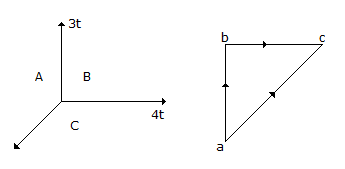# Civil Engineering - UPSC Civil Service Exam Questions - Discussion

16.

In the force system shown in the figure, the force CA should be[A]. 5 t [B]. - 5 t [C]. 4 t [D]. - 4 t

Explanation:

No answer description available for this question.

 Chnadu said: (Apr 1, 2014) 3^2+4^2 = 25. %25 = 5. And the direction same as shown.

 Arbina said: (Jul 16, 2014) 3^2+4^2 = SQUARE OF ANSWER. 25 = SQUARE OF ANSWER. TAKING SQUARE ROOT ON BOTH SIDE SO, 5 = ANSWER.

 Logu said: (Dec 9, 2014) This formula is used for center line marking.

 Asaithambi said: (Dec 14, 2016) Resultant force = ((horizontal force)^2 + (vertical force)^2))^1/2. = ((4t^2) + (3t^2))^1/2. = 5t.

 Zinabe Molla said: (Sep 14, 2017) Resultant force = ((horizontal force)^2 + (vertical force)^2))^1/2. = ((4t^2) + (3t^2))^1/2. = 5t.

 Saurabh Kadam said: (May 22, 2020) Is there fixed formula for resultant force?